# How to compare two cells in Excel (string comparison)

The tutorial shows how to compare text strings in Excel for case-insensitive and exact match. You will learn a number of formulas to compare two cells by their values, string length, or the number of occurrences of a specific character, as well as how to compare multiple cells.

When using Excel for data analysis, accuracy is the most vital concern. Incorrect information leads to missed deadlines, misjudged trends, wrong decisions and lost revenues.

While Excel formulas are always perfectly true, their results may be wrong because some flawed data penetrated into the system. In this case, the only remedy is to check data for accuracy. It's no big deal to compare two cells manually, but it's next to impossible to spot the differences between hundreds and thousands of text strings.

This tutorial will teach you how to automate the tedious and error-prone task of cell comparison and what formulas are best to use in each particular case.

## How to compare two cells in Excel

There are two different ways to compare strings in Excel depending on whether you seek case-sensitive or case-insensitive comparison.

### Case-insensitive formula to compare 2 cells

To compare two cells in Excel ignoring case, use a simple formula like this:

`=A1=B1`

Where A1 and B1 are the cells you are comparing. The result of the formula are Boolean values TRUE and FALSE.

If you want to output your own texts for matches and differences, embed the above statement in the logical test of the IF function. For example:

`=IF(A1=B1, "Equal", "Not equal")`

As you see in the screenshot below, both formulas compare text strings, dates and numbers equally well: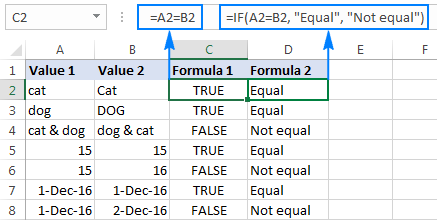### Case-sensitive formula to compare strings in Excel

In some situations, it may be important not only to compare text in two cells, but also to compare the character case. Case-sensitive text comparison can be done using the Excel EXACT function:

EXACT (text1, text2)

Where text1 and text2 are the two cells you are comparing.

Assuming your strings are in cells A2 and B2, the formula goes as follows:

`=EXACT(A2, B2)`

As the result, you get TRUE for text strings match exactly including the case of each character, FALSE otherwise.

If you want the EXACT function to deliver some other results, embed it in an IF formula and type your own text for value_if_true and value_if_false arguments:

`=IF(EXACT(A2 ,B2), "Exactly equal", "Not equal")`

The following screenshot shows the results of the case-sensitive string comparison in Excel: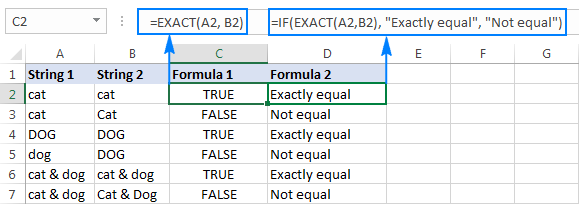## How to compare multiple cells in Excel

To compare more than 2 cells in a row, use the formulas discussed in the above examples in combination with the AND operator. The full details follow below.

### Case-insensitive formula to compare more than 2 cells

Depending on how you want to display the results, utilize one of the following formulas:

`=AND(A2=B2, A2=C2)`

or

`=IF(AND(A2=B2, A2=C2), "Equal", "Not equal")`

The AND formula returns TRUE if all of the cells contain the same value, FALSE if any value is different. The IF formula outputs the labels that you type in it, "Equal" and "Not equal" in this example.

As demonstrated in the screenshot below, the formula works perfectly with any data types - text, dates and numeric values: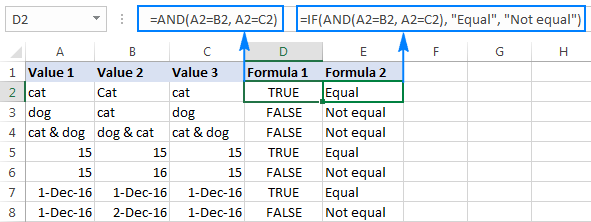### Case-sensitive formula to compare text in several cells

To compare multiple strings to each other to see if they match exactly, use the following formulas:

`=AND(EXACT(A2,B2), EXACT(A2, C2))`

Or

`=IF(AND(EXACT(A2,B2), EXACT(A2, C2)),"Exactly equal", "Not equal")`

Like in the previous example, the first formula delivers TRUE and FALSE values, whereas the second one displays your own texts for matches and differences: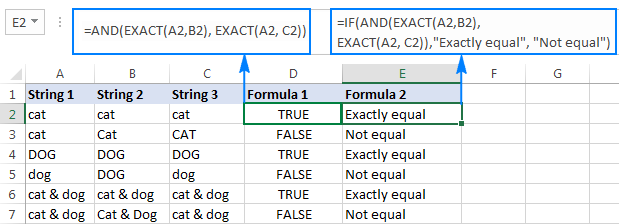## Compare a range of cells to a sample cell

The following examples show how you can verify that all cells in a given range contain the same text as in a sample cell.

### Case-insensitive formula to compare cells to a sample text

If the character case does not really matter, you can use the following formula to compare cells to a sample:

ROWS(range)*COLUMNS(range)=COUNTIF(range, sample cell)

In the logical test of the IF function, you compare two numbers:

• The total number of cells in a specified range (the number of rows multiplied by the number of columns), and
• The number of cells containing the same value as in the sample cell (returned by the COUNTIF function).

Assuming the sample text is in C2 and the strings to compare are in the range A2:B6, the formula goes as follows:

`=ROWS(A2:B6)*COLUMNS(A2:B6)=COUNTIF(A2:B6,C2)`

To make the results more user-friendly, i.e. output something like "All match" and "Not all match" instead of TRUE and FALSE, use the IF function like we did in the previous examples:

`=IF(ROWS(A2:B6)*COLUMNS(A2:B6)=COUNTIF(A2:B6,C2),"All match", "Not all match")`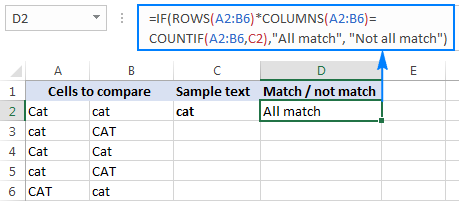As shown the above screenshot, the formula perfectly copes with a range of text strings, but it can also be used to compare numbers and dates.

### Case-sensitive formula to compare strings to a sample text

If the character case makes a difference, you can compare strings to the sample text using the following array formulas.

IF(ROWS(range)*COLUMNS(range)=SUM(--EXACT(sample_cell, range)), "text_if_match", "text_if_not match")

With the source range residing in A2:B6 and the sample text in C2, the formula takes the following shape:

`=IF(ROWS(A2:B6)*COLUMNS(A2:B6)=SUM(--EXACT(C2, A2:B6)), "All match", "Not all match")`

Unlike regular Excel formulas, array formulas are completed by pressing Ctrl + Shift + Enter. If entered correctly, Excel encloses the array formula in {curly braces}, as shown in the screenshot: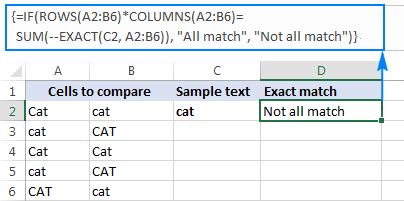## How to compare two cells by string length

Sometimes you may want to check if the text strings in each row contain an equal number of characters. The formula for this task is very simple. First, you get the string length of two cells using the LEN function, and then compare the numbers.

Supposing the strings to be compared are in cells A2 and B2, use either of the following formulas:

`=LEN(A2)=LEN(B2)`

Or

`=IF(LEN(A2)=LEN(B2), "Equal", "Not equal")`

As you already know, the first formula returns Boolean values TRUE or FALSE, whereas the second formula outputs your own results: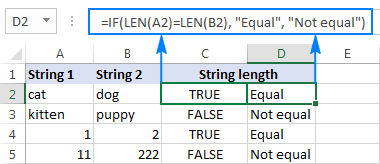As demonstrated in the screenshot above, the formulas work for text strings as well as numbers.

Tip. If two seemingly equal strings return different lengths, most likely the problem is in leading or trailing spaces in one or both cells. In this case, remove extra spaces using the TRIM function. The detailed explanation and formula examples can be found here: How to trim spaces in Excel.

## Compare two cells by occurrences of a specific character

This is the last example in our Excel Compare Strings tutorial, and it shows a solution for a rather specific task. Supposing, you have 2 columns of text strings that contain a character important to you. Your goal is to check whether two cells in each row contain the same number of occurrences of a given character.

To make things clearer, consider the following example. Let's say, you have two lists of orders shipped (column B) and received (column C). Each row contains orders for a specific item, whose unique identifier is included in all order IDs and is listed in the same row in column A (please see the screenshot below). You want to make sure that each row contains an equal number of shipped and received items with that specific ID.

To solve this problem, write a formula with the following logic.

• Firstly, replace the unique identifier with nothing using the SUBSTITUTE function:

`SUBSTITUTE(A1, character_to_count,"")`

• Then, calculate how many times the unique identifier appears in each cell. For this, get the string length without the unique identifier and subtract it from the total length of the string. This part shall be written for cell 1 and cell 2 individually, for example:

`LEN(cell 1) - LEN(SUBSTITUTE(cell 1, character_to_count, ""))`
and
`LEN(cell 2) - LEN(SUBSTITUTE(cell 2, character_to_count, ""))`

• Lastly, you compare these 2 numbers by placing the equality sign (=) in between the above parts.
LEN(cell 1) - LEN(SUBSTITUTE(cell 1, character_to_count, ""))=
LEN(cell 2) - LEN(SUBSTITUTE(cell 2, character_to_count, ""))

In our example, the unique identifier is in A2, and the strings to compare are in cells B2 and C2. So, the complete formula is as follows:

`=LEN(B2)-LEN(SUBSTITUTE(B2,\$A2,""))=LEN(C2)-LEN(SUBSTITUTE(C2,\$A2,""))`

The formula returns TRUE if cells B2 and C2 contain an equal number of occurrences of the character in A2, FALSE otherwise. To make the results more meaningful for your users, you can embed the formula in the IF function:

`=IF(LEN(B2)-LEN(SUBSTITUTE(B2, \$A2,""))=LEN(C2)-LEN(SUBSTITUTE(C2, \$A2,"")), "Equal", "Not equal")`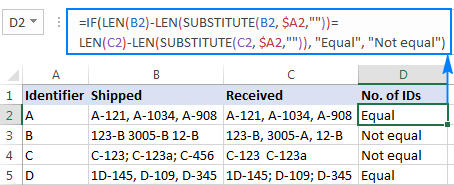As you can see in the screenshot above, the formula works perfectly despite a couple of additional complications:

• The character to be counted (unique identifier) can appear anywhere in a text string.
• The strings contain a variable number of characters and different separators such as semicolon, comma or space.

This is how you compare strings in Excel. To have a closer look at the formulas discussed in this tutorial, you are welcome to download Excel Compare Strings Worksheet. I thank you for reading and hope to see you on our blog next week.

## You may also be interested in

### 27 responses to "How to compare two cells in Excel (string comparison)"

1.Dave says:

This site is great. I was wondering if you could start posting some blogs about VBA coding and automating standard work tasks to save time.

2.Pankaj Chauhan says:

Your posts are awesome and explanatory enough that even a novice can understand typical formulas.

3.IRSHAD says:

hi Svetlana,
first of all, its highly appciable what you are doing for a newcomer in excel like me. i read all of your post but couldn't find what i am looking for so here I am giving details of my query and hope you will reply as you get time.

A B Result
0.481 0.25 High or 0.23 down sign
0.618 0 Less

Is it possible to use formulae to compare the two column and based on the column A shows the diffrence with color higlight and symbol of up and down.

thanks

•Svetlana Cheusheva (Ablebits.com Team) says:

You can calculate the difference with this simple formula: =B2-A2

Then, enter the following formula in column D. It returns 2 if B2 is greater then A2, 1 if B2 is equal to A2, and 0 if B2 is less than A2:

=IF(B2>A2, 2, IF(B2<A2, 0, 1))

Finally, create an icon set rule for column D like shown in the screenshot below. Please pay attention that the "Show icon only" box should be selected:4.pawan arya says:

Plz solving hlookup formula and send my email hlookup sheet

5.Bhaktha Singh J says:

Hi Svetlana,

I have columns of information as follows.
A:F
Row 1: A B C X X X
Row 2: A B X X X X
H:M
Row 1: E F G X X X
Row 2: E F X X X X

I want P:U to look as below.
Row 1: A B C D E F G
Row 2: A B E F

I have more than two thousand rows like this.

Regards,
Jeb Singh

6.Nafisa Fatema says:

Hi Svetlana,
I am trying to make a daily working record for our project. I need to have four column "time-in"; "time-out", "Total hours worked", "Overtime calculation". I can't seem to format the time-in , time-out cell to format such an 1.30am. For though I am selecting the option; when I enter the time after formatting the cells it shows the following format 1/8/1900 12:00:00 AM. By the way I am using MS Office 2016.
Thank you
Nafisa

7.HUZEFA KUKSHI says:

i want to manage my stock i given to dealer A 3 mobile which serial 123,124,125 and give 3 mobile to another dealer B which serial 126,127,128 now if dealer A sold 126 serial mobile than value return with false and if he sold 123 than value show as true because 123 serial is in stock of dealer A

8.Mrinal Singh says:

Hi

I wanted to give marks in a question according to the following scheme. It's a multiple choice problem with more than one option possible. There are 4 options. If a student gets it completely correct, he/she gets 4 marks. If he/she gets it partially correct, then marks are given according to the number of options which are correct for the student. But if even one option given is wrong, then -2 marks are awarded.
Ex: Let the answer be ABC. Then marks awarded according to answer given:
A: 1; B: 1; AB: 2; ABC: 4; D: -2; ABD: -2

How can we do its programming in excel?
Thanks

9.Mrinal Singh says:

Hi

I wanted to give marks in a question according to the following scheme. It's a multiple choice problem with more than one option possible. There are 4 options. If a student gets it completely correct, he/she gets 4 marks. If he/she gets it partially correct, then marks are given according to the number of options which are correct for the student. But if even one option given is wrong, then -2 marks are awarded.
Ex: Let the answer be ABC. Then marks awarded according to answer given:
A: 1; B: 1; AB: 2; ABC: 4; D: -2; ABD: -2

How can we do its programming in excel?
Thanks
MS

10.Nitika says:

Hi Svetlana, I have data in three columns in the pivot. Please see row 4 and 5, they are the same record but because of comma it is showing up in two different lines, is there is any fast way to clean such data?

GENEVA, TOWN OF-WTP NY 14456
GENOA, CITY OF IL 60135
GEORGE, CITY OF IA 51237
4.GEORGETOWN TOWN OF DE 19947
GEORGETOWN, CITY OF SC 29440
5.GEORGETOWN, TOWN OF DE 19947

11.Sanv says:

I'm looking for comparision of two cells by ignoring return chars.
For ex. when we compare below 2 values, it should return true.
I saw Exact, Using IF, TRIM but couldn't find how to ignore return chars

1. Hello World
2. Hello
World

12.Leah says:

If B2 is than or = to 100% of D2 I want the color to be green.
For the life of me and much time I can't figure this conditional format out.

13.Sijo says:

Hi Svetlana,

The training lesson is very useful. great help indeed. Thank you for putting up such wonderful lessons.

14.Joao says:

Hello Svetlana,

If column A has a word or sentence
And column B has another word or sentence

How can column C tell if column A and column B has at least a X equal letters in sequence that are the same, disconsidering capital or lower case sensitive

Example:

Cell A1 has word "daily"
Cell B1 has word "day"

So if X=2, means it will compare if at least 2 same letters in sequence appear in both cells of the same line.

In this case answer is yes because "daily" in cell A1 has the letters "da" and "day" in cell B1 also has the letters "da".

But in this same case if we program X=3, then it will come as No Equal 3 letters in a sequence, because "dai" is different than "day".

If you could help which formula to use would be greatly appreciate.

Thank you

•Gennady Terekhov (Ablebits.com Team) says:

Hello,

I'm afraid there's no easy way to solve your task with a formula. Using a VBA macro would be the best option here.

However, since we do not cover the programming area (VBA-related questions), I can advice you to try and look for the solution in VBA sections on mrexcel.com or excelforum.com.

Sorry I can't assist you better.

15.Toby says:

Hi,

I would like to ask if there is a formula or way we can count the number of words in a text in a cell and compare it to another cell and check if what words does not match.

16.sathi says:

how to prepare a database and then matching a digit to the database

17.Vinay Jain. says:

18.Murtaza Patrawala (India) says:

Thank you very much it was really helpful.

19.peter says:

Hello - Column A has some records with "syr.edu" as part of the text, and some do not. I want to use an IF statement in Column B to return a 1 if the record in Column A does include that text, and a 0 if the record in Column A doesn't include that text. I cannot find a way to structure it to look for just that portion of text, then return the 1 or 0.
Any suggestions?
Appreciated!
Peter

20.Ems says:

Given :
Cell A = ABACA, EUGENE FORNASA
Cell B = ABACA, EUGENE F.
How to check if Cell A = cell B
= In human decision Cell A is equal to Cell B, but in Excel this is not equal. Now how to check the content that is more or less the same

21.r10281 says:

How can we get this?
column A1 = Springseason
column B1 = Spring Season
Result C1 = Spring Season

•Alexander Trifuntov (Ablebits.com Team) says:

Hello!
If I understand your task correctly, the following formula should work for you:

=IF(SUBSTITUTE(B1," ","")=A1,B1,A1)

22.JAYAKUMAR PANICKER says:

Hai...
I want to compare 80 Answers of an MCQ as String Comparison and get the Counts of "True" Result. I got it as following:
I. =EXACT(F2,F3) / and copying the result till CG4.
II.=COUNTIF(F3:CG3, TRUE)
I would like to know, if I can get the number of "True" Results; using a SINGLE FORMULA.
Thank you.